# Class 9 RD Sharma Solutions – Chapter 8 Introduction to Lines and Angles- Exercise 8.2 | Set 2

• Last Updated : 04 May, 2021

### Question 11. In Figure, ACB is a line such that ∠DCA = 5x and ∠DCB = 4x.  Find the value of x.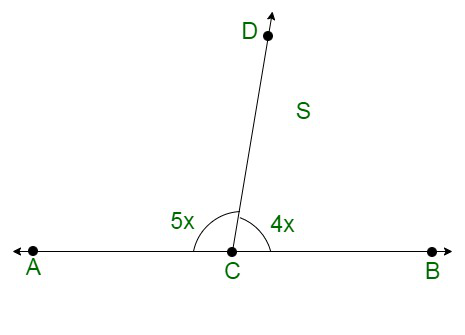Solution:

Attention reader! All those who say programming isn't for kids, just haven't met the right mentors yet. Join the  Demo Class for First Step to Coding Coursespecifically designed for students of class 8 to 12.

The students will get to learn more about the world of programming in these free classes which will definitely help them in making a wise career choice in the future.

It is given that ACB is a line in the figure given below.

Thus, ∠ACD and ∠BCD form a linear pair.

Therefore, their sum must be equal toh 180°.

Or we can say that

∠ACD + ∠BCD = 180°

Also, ∠ACD = 4x and ∠BCD = 5x.

This further simplifies to:

4x + 5x = 180

9x = 180

x =x = 20°

### Question 12. In the given figure, ∠POR = 3x and ∠QOR = 2x + 10, find the value of x for which POQ will be a line.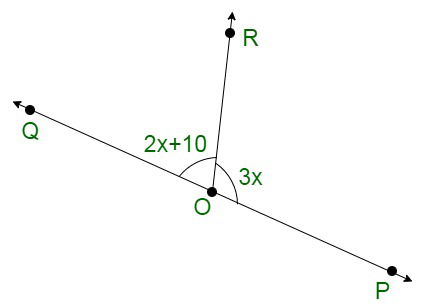Solution:

Here we have POQ as a line

So, ∠POR and ∠QOR form a linear pair.

Therefore, their sum must be equal to 180°

Or

∠POR + ∠QOR = 180°

It is given that ∠POR = (3x)° and ∠QOR = (2x + 10)°. On substituting these values above we get,

3x + (2x + 10) = 180°

3x + 2x + 10 = 180°

5x + 10 = 180°

5x = 180 – 10

5x = 170

x =x = 34°

### Question 13. In the given figure, a is greater than b by one-third of a right-angle. Find the values of a and b.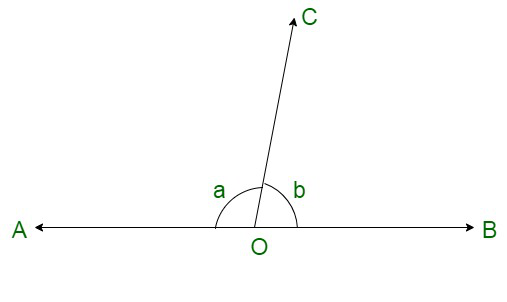Solution:

It is given that in the figure given below; a is greater than b by one-third of a right angle.

Or we can say that, the difference between a and b isi.e.

a – b =a – b = 30° ……..(i)

Also a and b forn a linear pair. Therefore, their sum must be equal to 180°.

We can say that:

a + b = 180° ……….(ii)

On adding (i) and (ii), we get:

2a = 180 + 30

2a = 210

a =a = 105°

On putting a = 105 in (i)

105 – b = 30

-b = 30 – 105

-b = -75

b = 75°

Hence, a = 105° and b = 75°

### Question 14. What value of y would make AOB a line in the given figure, if ∠AOC = 4y and ∠BOC = (6y + 30)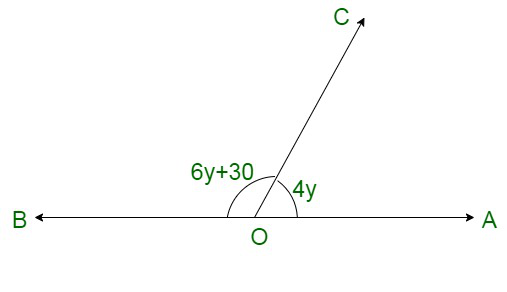Solution:

Let us assume, AOB as a straight line.

This makes ∠AOC and ∠BOC  to form a linear pair. Therefore, their sum must be equal to 180°.

We can say that:

∠AOC + ∠BOC = 180°

Also, ∠AOC = 4y  and ∠BOC = 6y + 30. This further simplifies to:

4y + (6y + 30) = 180

10y + 30 = 180

10y = 180 – 30

10y = 150

y =y = 15°

Thus, the value of y = 15° make AOB as a line.

### Question 15. If the given figure, ∠AOF and ∠FOG form a linear pair.

∠EOB = ∠FOC = 90° and ∠DOC = ∠FOG = ∠AOB = 30°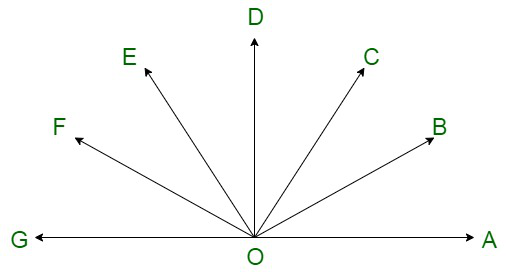(i) Find the measure of ∠FOE, ∠COB and ∠DOE.

(ii) Name all the right angles.

(iii) Name three pairs of adjacent complementary angles.

(iv) Name three pairs of adjacent supplementary angles.

(v) Name three pairs of adjacent angles.

Solution:

The given figure is as follows:

(i) It is given that ∠AOB, ∠FOE, ∠EOB and ∠FOG form a linear pair.

Therefore, their sum must be equal to 180°

i.e.

∠AOB + ∠FOE + ∠EOB + ∠FOG = 180°

It is given that:

∠FOG = 30°

∠AOB = 30°

∠EOB = 90° in equation above, we get:

∠AOB + ∠FOE + ∠EOB + ∠FOG = 180°

30° + ∠FOE + 90° + 30° = 180°

∠FOE + 150° = 180°

∠FOE = 180° – 150°

∠FOE = 30°

It is given that

∠FOC = 90°

From the above figure:

∠FOE + ∠DOE + ∠COD = 90°

30° + ∠DOE + 30° = 90°

∠DOE + 60° = 90°

∠DOE = 90° – 60°

∠DOE = 30°

Similarly, we have:

∠EOB = 90°

From the above figure:

∠DOE + ∠DOC + ∠COB = 90°

30° + 30° + ∠COB = 90°

∠COB + 60° = 90°

∠COB = 90° – 60°

∠COB = 30°

(ii) We have:

∠FOG = 30°

∠FOE = 30°

∠EOD = 30°

∠COD = 30°

∠COB = 30°

∠AOB = 30°

From the figure above and the measurements of the calculated angles we get two right angles as ∠DOG and ∠AOD.

Two right angles are already given as ∠FOC and ∠EOB

(iii) We have to find the three pair of adjacent complementary angles.

We know that ∠EOB is a right angle.

Therefore,

∠EOC and ∠COB are complementary angles.

Similarly, ∠AOD is a right angle.

Therefore,

∠AOC and ∠COD are complementary angles.

(iv) We have to find the three pair of adjacent supplementary angles.

Since, ∠AOG is a straight line.

Therefore, following are the three linear pair, which are supplementary:

∠AOB and ∠BOG

∠AOC and ∠COG

∠AOD and ∠DOG

(v) We have to find pair of adjacent angles, which are as follows:

∠AOB and ∠BOC

∠COD and ∠DOE

∠EOF and ∠FOG

### Question 16. In the given figure, OP, OQ , OR, and OS are four rays. Prove that:

∠POQ + ∠QOR + ∠SOR + ∠POS = 360°.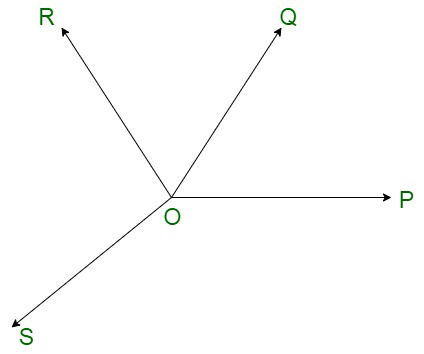Solution:

Let us draw TOP as a straight line.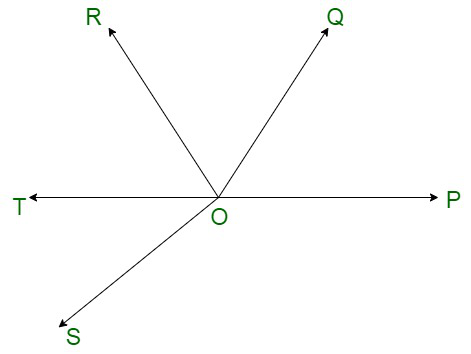Since, TOP is a line, therefore, ∠POQ, ∠QOR and ∠ROT form a linear pair.

Also, ∠POS and ∠SOT form a linear pair.

Thus, we have:

∠POQ + ∠QOR + ∠ROT = 180° ……(i)

and

∠POS + ∠SOT = 180°  …….(ii)

On adding (i) and (ii), we get;

(∠POQ + ∠QOR + ∠ROT) + (∠POS + ∠SOT) = 180° + 180°

∠POQ + ∠QOR + (∠ROT + ∠SOT) + ∠POS = 360°

∠POQ + ∠QOR + ∠SOR + ∠POS = 360°

Hence proved.

### Question 17. In the given figure, ray OS stand on a line POQ, Ray OR and ray OT are angle bisectors of ∠POS and∠SOQ respectively. If ∠POS = x, find ∠ROT.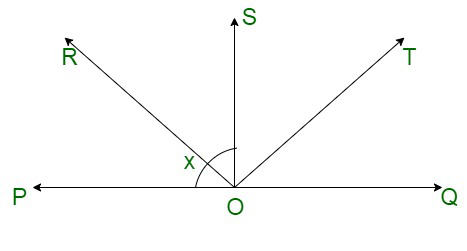Solution:

In the figure given below, we have

Ray OR as the bisector of ∠POS

Therefore,

∠POR = ∠ROS

or,

∠POS = 2∠ROS ………..(i)

Similarly, ray OT as the bisector of ∠SOQ

Therefore,

∠TOQ = ∠TOS

or,

∠QOS = 2∠TOS ……….(ii)

Also, Ray OS stand on a line POQ. Therefore, ∠POS and ∠QOS form a linear pair.

Thus,

∠POS + ∠QOS = 180°

From (i) and (ii)

2∠ROS + 2∠TOS = 180°

2(∠ROS + ∠TOS) = 180°

∠ROS + ∠TOS =∠ROT = 90°

### Question 18. In the given figure, lines PQ and RS intersect each other at point O. If ∠POR: ∠ROQ = 5:7, find all the angles.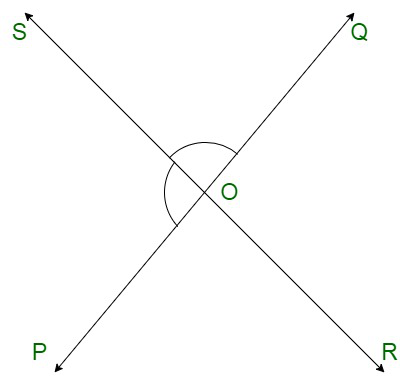Solution:

Let ∠POR and ∠ROQ be 5x and 7x respectively.

Since, Ray OR stand on line POQ. Thus, ∠POR and ∠ROQ form a linear pair.

Therefore, their sum must be equal to 180°.

Or,

∠POR + ∠ROQ = 180°

5x + 7x = 180°

12x = 180°

x =x = 15° …….(i)

Thus,

∠POR = 5x

= 5(15)

= 75

∠POR = 75°

Thus,

∠ROQ = 7x

= 7(15)

=105

∠ROQ = 105°

It is evident from the figure, that ∠QOS and ∠POR are vertically opposite angles.

And we know that vertically opposite angles are equal.

Therefore,

∠QOS = ∠POR

∠QOS = 75°

Similarlly, ∠POS and ∠ROQ are vertically opposite angles.

And we know that vertically opposite angles are equal.

Therefore,

∠POS = ∠ROQ

∠POS = 105°

### Question 19. In the given figure, POQ is a line. Ray OR is perpendicular to line PQ. OS is another ray lying between rays OP and OR. Prove that

∠ROS = 1212 (∠QOS − POS).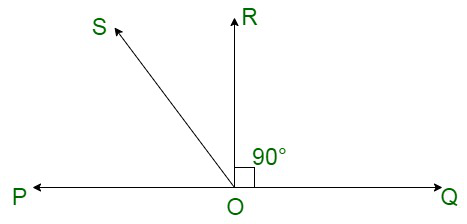Solution:

The given figure shows:

We have POQ as a line. Ray OR is perpendicular to line PQ. Therefore,

∠ROQ = 90°

∠POR = 90°

From the figure above, we get

∠ROS + ∠POS = 90° ………(i)

∠POS and ∠QOS form a linear pair.

Therefore,

∠QOS + ∠POS = 180°   ……(ii)

From (i) and (ii) equation we get:

∠QOS + ∠POS = 2 × 90 ∠QOS + ∠POS = 2 × 90

∠QOS + ∠POS = 2(∠ROS + ∠POS)

2∠ROS = ∠QOS – ∠POS

∠ROS =(∠QOS – ∠POS)

Hence, proved.

My Personal Notes arrow_drop_up